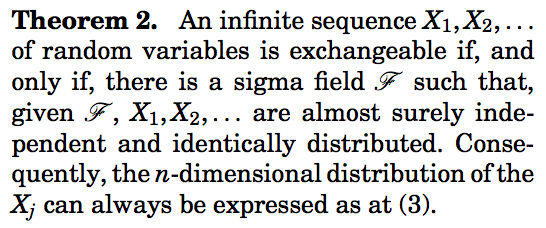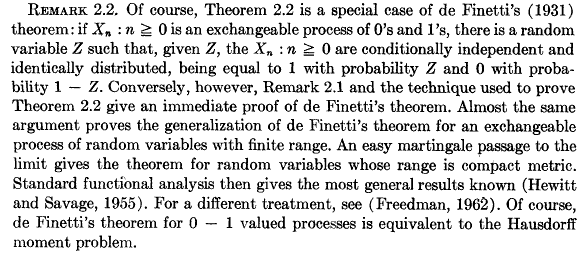# Exchangeability, credit risk and risk measures

Exchangeability is an extremely concept, since (most of the time) analytical expressions can be derived. But it can also be used to observe some unexpected behaviors, that we will discuss later on with a more general setting. For instance, in a old post, I discussed connexions between correlation and risk measures (using simulations to illustrate, but in the context of exchangeable risk, calculations can be performed more accurately). Consider again the standard credit risk problem, where the quantity of interest is the number of defaults in a portfolio. Consider an homogeneous portfolio of exchangeable risk. The quantity of interest is hereor perhaps the quantile function of the sum (since the Value-at-Risk is the standard risk measure). We have seen yesterday that – given the latent factor –(either the company defaults, or not), so thati.e. we can derive the (unconditional) distribution of the sumso that the probability function of the sum is, assuming thatThus, the following code can be used to calculate the quantile function

```> proba=function(s,a,m,n){
+ b=a/m-a
+ choose(n,s)*integrate(function(t){t^s*(1-t)^(n-s)*
+ dbeta(t,a,b)},lower=0,upper=1,subdivisions=1000,
+ stop.on.error =  FALSE)\$value
+ }
> QUANTILE=function(p=.99,a=2,m=.1,n=500){
+ V=rep(NA,n+1)
+ for(i in 0:n){
+ V[i+1]=proba(i,a,m,n)}
+ V=V/sum(V)
+ return(min(which(cumsum(V)>p))) }```

Now observe that since variates are exchangeable, it is possible to calculate explicitly correlations of defaults. Herei.e.Thus, the correlation between two default indicators is thenUnder the assumption that the latent factor is beta distributedwe getThus, as a function of the parameter of the beta distribution (we consider beta distributions with the same mean, i.e. the same margin distributions, so we have only one parameter left, with is simply the correlation of default indicators), it is possible to plot the quantile function,

```> PICTURE=function(P){
+ A=seq(.01,2,by=.01)
+ VQ=matrix(NA,length(A),5)
+ for(i in 1:length(A)){
+ VQ[i,1]=QUANTILE(a=A[i],p=.9,m=P)
+ VQ[i,2]=QUANTILE(a=A[i],p=.95,m=P)
+ VQ[i,3]=QUANTILE(a=A[i],p=.975,m=P)
+ VQ[i,4]=QUANTILE(a=A[i],p=.99,m=P)
+ VQ[i,5]=QUANTILE(a=A[i],p=.995,m=P)
+ }
+ plot(A,VQ[,5],type="s",col="red",ylim=
+ c(0,max(VQ)),xlab="",ylab="")
+ lines(A,VQ[,4],type="s",col="blue")
+ lines(A,VQ[,3],type="s",col="black")
+ lines(A,VQ[,2],type="s",col="blue",lty=2)
+ lines(A,VQ[,1],type="s",col="red",lty=2)
+ lines(A,rep(500*P,length(A)),col="grey")
+ legend(3,max(VQ),c("quantile 99.5%","quantile 99%",
+ "quantile 97.5%","quantile 95%","quantile 90%","mean"),
+ col=c("red","blue","black",
+"blue","red","grey"),
+ lty=c(1,1,1,2,2,1),border=n)
+}```

e.g. with a (marginal) default probability of 15%,

`> PICTURE(.15)`On this graph, we observe that the stronger the correlation (the more on the left), the higher the quantile… Note that the same graph can be plotted with on the X-axis the correlation,Which is quite intuitive, somehow. But if the marginal probability of default decreases, increasing the correlation might decrease the risk (i.e. the quantile function),

`> PICTURE(.05)`(with the modified code to visualize the quantile as a function of the underlying default correlation) or even worse,

`> PICTURE(.0075)`And it because all the more counterintuitive that the default probability decreases ! So in the case of a portfolio of non-very risky bond issuers (with high ratings), assuming a very strong correlation will lower risk based capital !

# de Finetti’s theorem and exchangeability

This week, we will start to work on multivariate models, and non-independence. The first idea to discuss non-independence will be to use the concept ofexchangeability. A sequence of random variableis said to be exchangeable if for allfor any permutationof. A standard example is the case where, withandSince, a necessary condition is thati.e.Since this inequality should hold for allit comes that necessarily.
de Finetti (1931): Letbe a sequence of random variables with values inis exchangeable if and only if there exists a distribution functiononsuch thatwhere. Note thatis the distribution function of random variableA nice proof of that result can be found in Heath & Sudderth (1995) – see alsoSchervish (1995)Chow & Teicher (1997) or Durrett (2010) and also probably in several bayesian books because that result has a strong interpretation in bayesian inference (as far as I understood, see e.g. Jaynes (1982)).
From the exchangeability condition, for any permutationof,that can be inverted inThe idea is then to extend the size of the vector, i.e. for all, defineso that, if we condition on,but since given the sum of components of, all possible rearrangements of the ones among theelements are equally likely, we can writeThe first idea is to work on the blue term, and to invocate a theorem of approximation of the hypergeometric distributionto a binomial distribution, whenbecomes large. ThenLetand letdenote the cumulative distribution function of.The idea is then to write the sum as an integral, with respect to that distribution,The theorem is then obtained since, i.e.In the case of non-binary sequences, there is an extension of the previous result,
Hewitt & Savage (1955): Letbe a sequence of random variables with values in.is exchangeable if and only if there exists a measureonsuch thatwhereis the measure associated to the empirical measureandFor instance, in the Gaussian case mentioned earlier, ifthenwherei.e. conditionally on, theare conditionally independent, with distribution. The proof can be found in Kingman (1978) and is based on martingale arguments.
Note that in the Gaussian case,whereare i.i.d. random variables. To go further on exchangeability and related topics, see Aldous (1985)  (see also here).
This construction can be used in credit risk, to model defaults in an homogeneous portfolio, see e.g. Frey (2001),Assuming a Beta distribution for the latent factor, we can derive the probability distribution of the sumSinceif we assume that – given the latent factor –(either the company defaults, or not),i.e.Thus, we can derive the (unconditional) distribution of the sumi.e.```> proba=function(s,a,m,n){
+ b=a/m-a
+ choose(n,s)*integrate(function(t){t^s*(1-t)^(n-s)*
+ dbeta(t,a,b)},lower=0,upper=1)\$value
+ }```

Based on that function, it is possible to plot the probability distribution over. In the upper corner is plotted the density of the Beta distribution.

```> a=2
> m=.2
+ n=10
+ V=rep(NA,n+1)
+ for(i in 0:n){
+ V[i+1]=proba(i,a,m,n)}
> barplot(V,names.arg=0:10)```Those two theorems are extremely close,

De Finetti’s theorem: a random sequenceofrandom variables is exchangeable if and only if‘s are conditionnally independent, conditionnally on some random variable.

Hewitt-Savage’s theorem: a random sequenceis exchangeable if and only if‘s are conditionnally independent, conditionnally on some sigma-algebraOlshen (1974), proposed an interesting discussion about those theorems, see also in the Encyclopedia of Statistical Science,The subtle difference between those two theorem is also discussed in Freedman (1965)